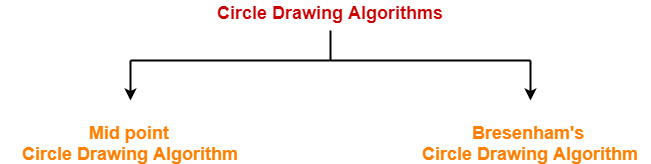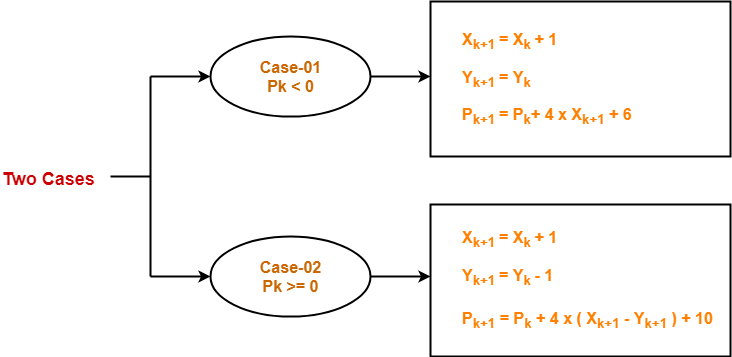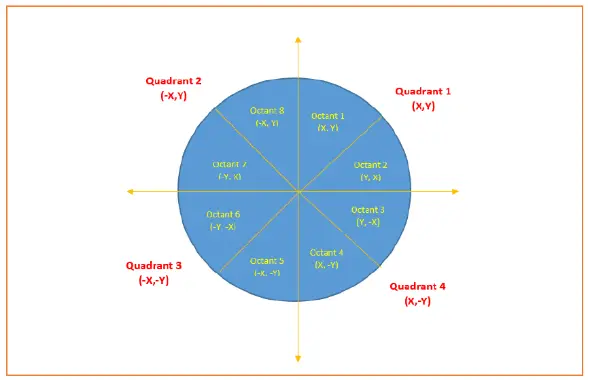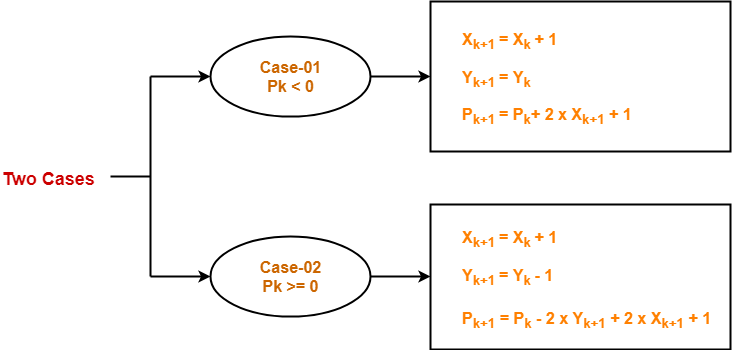## Circle Drawing Algorithms-

In computer graphics, popular algorithms used to generate circle are-1. Mid Point Circle Drawing Algorithm
2. Bresenham Circle Drawing Algorithm

## Bresenham Circle Drawing Algorithm-

 Given the centre point and radius of circle,Bresenham Circle Drawing Algorithm attempts to generate the points of one octant.

The points for other octacts are generated using the eight symmetry property.

Also Read- Mid Point Circle Drawing Algorithm

### Procedure-

Given-

• Centre point of Circle = (X0, Y0)
• Radius of Circle = R

The points generation using Bresenham Circle Drawing Algorithm involves the following steps-

### Step-01:

Assign the starting point coordinates (X0, Y0) as-

• X0 = 0
• Y0 = R

### Step-02:

Calculate the value of initial decision parameter P0 as-

P0 = 3 – 2 x R

### Step-03:

Suppose the current point is (Xk, Yk) and the next point is (Xk+1, Yk+1).

Find the next point of the first octant depending on the value of decision parameter Pk.### Step-04:

If the given centre point (X0, Y0) is not (0, 0), then do the following and plot the point-

• Xplot = Xc + X0
• Yplot = Yc + Y0

Here, (Xc, Yc) denotes the current value of X and Y coordinates.

### Step-05:

Keep repeating Step-03 and Step-04 until Xplot => Yplot.

### Step-06:

Step-05 generates all the points for one octant.

To find the points for other seven octants, follow the eight symmetry property of circle.

This is depicted by the following figure-## Problem-01:

Given the centre point coordinates (0, 0) and radius as 8, generate all the points to form a circle.

## Solution-

Given-

• Centre Coordinates of Circle (X0, Y0) = (0, 0)
• Radius of Circle = 8

### Step-01:

Assign the starting point coordinates (X0, Y0) as-

• X0 = 0
• Y0 = R = 8

### Step-02:

Calculate the value of initial decision parameter P0 as-

P0 = 3 – 2 x R

P0 = 3 – 2 x 8

P0 = -13

### Step-03:

As Pinitial < 0, so case-01 is satisfied.

Thus,

• Xk+1 = Xk + 1 = 0 + 1 = 1
• Yk+1 = Yk = 8
• Pk+1 = Pk + 4 x Xk+1 + 6 = -13 + (4 x 1) + 6 = -3

### Step-04:

This step is not applicable here as the given centre point coordinates is (0, 0).

### Step-05:

Step-03 is executed similarly until Xk+1 >= Yk+1 as follows-

 Pk Pk+1 (Xk+1, Yk+1) (0, 8) -13 -3 (1, 8) -3 11 (2, 8) 11 5 (3, 7) 5 7 (4, 6) 7 (5, 5) Algorithm TerminatesThese are all points for Octant-1.

Algorithm calculates all the points of octant-1 and terminates.

Now, the points of octant-2 are obtained using the mirror effect by swapping X and Y coordinates.

 Octant-1 Points Octant-2 Points (0, 8) (5, 5) (1, 8) (6, 4) (2, 8) (7, 3) (3, 7) (8, 2) (4, 6) (8, 1) (5, 5) (8, 0) These are all points for Quadrant-1.

Now, the points for rest of the part are generated by following the signs of other quadrants.

The other points can also be generated by calculating each octant separately.

Here, all the points have been generated with respect to quadrant-1-

 Quadrant-1 (X,Y) Quadrant-2 (-X,Y) Quadrant-3 (-X,-Y) Quadrant-4 (X,-Y) (0, 8) (0, 8) (0, -8) (0, -8) (1, 8) (-1, 8) (-1, -8) (1, -8) (2, 8) (-2, 8) (-2, -8) (2, -8) (3, 7) (-3, 7) (-3, -7) (3, -7) (4, 6) (-4, 6) (-4, -6) (4, -6) (5, 5) (-5, 5) (-5, -5) (5, -5) (6, 4) (-6, 4) (-6, -4) (6, -4) (7, 3) (-7, 3) (-7, -3) (7, -3) (8, 2) (-8, 2) (-8, -2) (8, -2) (8, 1) (-8, 1) (-8, -1) (8, -1) (8, 0) (-8, 0) (-8, 0) (8, 0) These are all points of the Circle.

## Problem-02:

Given the centre point coordinates (10, 10) and radius as 10, generate all the points to form a circle.

## Solution-

Given-

• Centre Coordinates of Circle (X0, Y0) = (10, 10)
• Radius of Circle = 10

### Step-01:

Assign the starting point coordinates (X0, Y0) as-

• X0 = 0
• Y0 = R = 10

### Step-02:

Calculate the value of initial decision parameter P0 as-

P0 = 3 – 2 x R

P0 = 3 – 2 x 10

P0 = -17

### Step-03:

As Pinitial < 0, so case-01 is satisfied.

Thus,

• Xk+1 = Xk + 1 = 0 + 1 = 1
• Yk+1 = Yk = 10
• Pk+1 = Pk + 4 x Xk+1 + 6 = -17 + (4 x 1) + 6 = -7

### Step-04:

This step is applicable here as the given centre point coordinates is (10, 10).

Xplot = Xc + X0 = 1 + 10 = 11

Yplot = Yc + Y0 = 10 + 10 = 20

### Step-05:

Step-03 and Step-04 are executed similarly until Xplot => Yplot as follows-

 Pk Pk+1 (Xk+1, Yk+1) (Xplot, Yplot) (0, 10) (10, 20) -17 -7 (1, 10) (11, 20) -7 7 (2, 10) (12, 20) 7 -7 (3, 9) (13, 19) -7 15 (4, 9) (14, 19) 15 13 (5, 8) (15, 18) 13 19 (6, 7) (16, 17) Algorithm TerminatesThese are all points for Octant-1.

Algorithm calculates all the points of octant-1 and terminates.

Now, the points of octant-2 are obtained using the mirror effect by swapping X and Y coordinates.

 Octant-1 Points Octant-2 Points (10, 20) (17, 16) (11, 20) (18, 15) (12, 20) (19, 14) (13, 19) (19, 13) (14, 19) (20, 12) (15, 18) (20, 11) (16, 17) (20, 10) These are all points for Quadrant-1.

Now, the points for rest of the part are generated by following the signs of other quadrants.

The other points can also be generated by calculating each octant separately.

Here, all the points have been generated with respect to quadrant-1-

 Quadrant-1 (X,Y) Quadrant-2 (-X,Y) Quadrant-3 (-X,-Y) Quadrant-4 (X,-Y) (10, 20) (10, 20) (10, 0) (10, 0) (11, 20) (9, 20) (9, 0) (11, 0) (12, 20) (8, 20) (8, 0) (12, 0) (13, 19) (7, 19) (7, 1) (13, 1) (14, 19) (6, 19) (6, 1) (14, 1) (15, 18) (5, 18) (5, 2) (15, 2) (16, 17) (4, 17) (4, 3) (16, 3) (17, 16) (3, 16) (3, 4) (17, 4) (18, 15) (2, 15) (2, 5) (18, 5) (19, 14) (1, 14) (1, 6) (19, 6) (19, 13) (1, 13) (1, 7) (19, 7) (20, 12) (0, 12) (0, 8) (20, 8) (20, 11) (0, 11) (0, 9) (20, 9) (20, 10) (0, 10) (0, 10) (20, 10) These are all points of the Circle.

## Advantages of Bresenham Circle Drawing Algorithm-

The advantages of Bresenham Circle Drawing Algorithm are-

• The entire algorithm is based on the simple equation of circle X2 + Y2 = R2.
• It is easy to implement.

## Disadvantages of Bresenham Circle Drawing Algorithm-

The disadvantages of Bresenham Circle Drawing Algorithm are-

• Like Mid Point Algorithm, accuracy of the generating points is an issue in this algorithm.
• This algorithm suffers when used to generate complex and high graphical images.
• There is no significant enhancement with respect to performance.

To gain better understanding about Bresenham Circle Drawing Algorithm,

Watch this Video Lecture

Get more notes and other study material of Computer Graphics.

Watch video lectures by visiting our YouTube channel LearnVidFun.

## Circle Drawing Algorithms-

In computer graphics, popular algorithms used to generate circle are-1. Mid Point Circle Drawing Algorithm
2. Bresenham’s Circle Drawing Algorithm

## Mid Point Circle Drawing Algorithm-

 Given the centre point and radius of circle,Mid Point Circle Drawing Algorithm attempts to generate the points of one octant.

The points for other octacts are generated using the eight symmetry property.

### Procedure-

Given-

• Centre point of Circle = (X0, Y0)
• Radius of Circle = R

The points generation using Mid Point Circle Drawing Algorithm involves the following steps-

### Step-01:

Assign the starting point coordinates (X0, Y0) as-

• X0 = 0
• Y0 = R

### Step-02:

Calculate the value of initial decision parameter P0 as-

P0 = 1 – R

### Step-03:

Suppose the current point is (Xk, Yk) and the next point is (Xk+1, Yk+1).

Find the next point of the first octant depending on the value of decision parameter Pk.### Step-04:

If the given centre point (X0, Y0) is not (0, 0), then do the following and plot the point-

• Xplot = Xc + X0
• Yplot = Yc + Y0

Here, (Xc, Yc) denotes the current value of X and Y coordinates.

### Step-05:

Keep repeating Step-03 and Step-04 until Xplot >= Yplot.

### Step-06:

Step-05 generates all the points for one octant.

To find the points for other seven octants, follow the eight symmetry property of circle.

This is depicted by the following figure-## Problem-01:

Given the centre point coordinates (0, 0) and radius as 10, generate all the points to form a circle.

## Solution-

Given-

• Centre Coordinates of Circle (X0, Y0) = (0, 0)
• Radius of Circle = 10

### Step-01:

Assign the starting point coordinates (X0, Y0) as-

• X0 = 0
• Y0 = R = 10

### Step-02:

Calculate the value of initial decision parameter P0 as-

P0 = 1 – R

P0 = 1 – 10

P0 = -9

### Step-03:

As Pinitial < 0, so case-01 is satisfied.

Thus,

• Xk+1 = Xk + 1 = 0 + 1 = 1
• Yk+1 = Yk = 10
• Pk+1 = Pk + 2 x Xk+1 + 1 = -9 + (2 x 1) + 1 = -6

### Step-04:

This step is not applicable here as the given centre point coordinates is (0, 0).

### Step-05:

Step-03 is executed similarly until Xk+1 >= Yk+1 as follows-

 Pk Pk+1 (Xk+1, Yk+1) (0, 10) -9 -6 (1, 10) -6 -1 (2, 10) -1 6 (3, 10) 6 -3 (4, 9) -3 8 (5, 9) 8 5 (6, 8) Algorithm TerminatesThese are all points for Octant-1.

Algorithm calculates all the points of octant-1 and terminates.

Now, the points of octant-2 are obtained using the mirror effect by swapping X and Y coordinates.

 Octant-1 Points Octant-2 Points (0, 10) (8, 6) (1, 10) (9, 5) (2, 10) (9, 4) (3, 10) (10, 3) (4, 9) (10, 2) (5, 9) (10, 1) (6, 8) (10, 0) These are all points for Quadrant-1.

Now, the points for rest of the part are generated by following the signs of other quadrants.

The other points can also be generated by calculating each octant separately.

Here, all the points have been generated with respect to quadrant-1-

 Quadrant-1 (X,Y) Quadrant-2 (-X,Y) Quadrant-3 (-X,-Y) Quadrant-4 (X,-Y) (0, 10) (0, 10) (0, -10) (0, -10) (1, 10) (-1, 10) (-1, -10) (1, -10) (2, 10) (-2, 10) (-2, -10) (2, -10) (3, 10) (-3, 10) (-3, -10) (3, -10) (4, 9) (-4, 9) (-4, -9) (4, -9) (5, 9) (-5, 9) (-5, -9) (5, -9) (6, 8) (-6, 8) (-6, -8) (6, -8) (8, 6) (-8, 6) (-8, -6) (8, -6) (9, 5) (-9, 5) (-9, -5) (9, -5) (9, 4) (-9, 4) (-9, -4) (9, -4) (10, 3) (-10, 3) (-10, -3) (10, -3) (10, 2) (-10, 2) (-10, -2) (10, -2) (10, 1) (-10, 1) (-10, -1) (10, -1) (10, 0) (-10, 0) (-10, 0) (10, 0) These are all points of the Circle.

## Problem-02:

Given the centre point coordinates (4, -4) and radius as 10, generate all the points to form a circle.

## Solution-

Given-

• Centre Coordinates of Circle (X0, Y0) = (4, -4)
• Radius of Circle = 10

As stated in the algorithm,

• We first calculate the points assuming the centre coordinates is (0, 0).
• At the end, we translate the circle.

Step-01, Step-02 and Step-03 are already completed in Problem-01.

Now, we find the values of Xplot and Yplot using the formula given in Step-04 of the main algorithm.

The following table shows the generation of points for Quadrant-1-

• Xplot = Xc + X= 4 + X0
• Yplot = Yc + Y0 = 4 + Y0

 (Xk+1, Yk+1) (Xplot, Yplot) (0, 10) (4, 14) (1, 10) (5, 14) (2, 10) (6, 14) (3, 10) (7, 14) (4, 9) (8, 13) (5, 9) (9, 13) (6, 8) (10, 12) (8, 6) (12, 10) (9, 5) (13, 9) (9, 4) (13, 8) (10, 3) (14, 7) (10, 2) (14, 6) (10, 1) (14, 5) (10, 0) (14, 4) These are all points for Quadrant-1.

The following table shows the points for all the quadrants-

 Quadrant-1 (X,Y) Quadrant-2 (-X,Y) Quadrant-3 (-X,-Y) Quadrant-4 (X,-Y) (4, 14) (4, 14) (4, -6) (4, -6) (5, 14) (3, 14) (3, -6) (5, -6) (6, 14) (2, 14) (2, -6) (6, -6) (7, 14) (1, 14) (1, -6) (7, -6) (8, 13) (0, 13) (0, -5) (8, -5) (9, 13) (-1, 13) (-1, -5) (9, -5) (10, 12) (-2, 12) (-2, -4) (10, -4) (12, 10) (-4, 10) (-4, -2) (12, -2) (13, 9) (-5, 9) (-5, -1) (13, -1) (13, 8) (-5, 8) (-5, 0) (13, 0) (14, 7) (-6, 7) (-6, 1) (14, 1) (14, 6) (-6, 6) (-6, 2) (14, 2) (14, 5) (-6, 5) (-6, 3) (14, 3) (14, 4) (-6, 4) (-6, 4) (14, 4) These are all points of the Circle.

## Advantages of Mid Point Circle Drawing Algorithm-

The advantages of Mid Point Circle Drawing Algorithm are-

• It is a powerful and efficient algorithm.
• The entire algorithm is based on the simple equation of circle X2 + Y2 = R2.
• It is easy to implement from the programmer’s perspective.
• This algorithm is used to generate curves on raster displays.

## Disadvantages of Mid Point Circle Drawing Algorithm-

The disadvantages of Mid Point Circle Drawing Algorithm are-

• Accuracy of the generating points is an issue in this algorithm.
• The circle generated by this algorithm is not smooth.
• This algorithm is time consuming.

### Important Points

• Circle drawing algorithms take the advantage of 8 symmetry property of circle.
• Every circle has 8 octants and the circle drawing algorithm generates all the points for one octant.
• The points for other 7 octants are generated by changing the sign towards X and Y coordinates.
• To take the advantage of 8 symmetry property, the circle must be formed assuming that the centre point coordinates is (0, 0).
• If the centre coordinates are other than (0, 0), then we add the X and Y coordinate values with each point of circle with the coordinate values generated by assuming (0, 0) as centre point.

To gain better understanding about Mid Point Circle Drawing Algorithm,

Watch this Video Lecture

Next Article- Bresenham Circle Drawing Algorithm

Get more notes and other study material of Computer Graphics.

Watch video lectures by visiting our YouTube channel LearnVidFun.